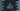# C program to print a new line without using \n# Print a new line without using \n :

Normally, we use \n in a printf statement to print one new line. This is the most commonly used method in the C programming language. We have a couple of other ways to print one new line in C without using \n. In this blog post, I will show you two different ways to do that. Let’s have a look :

## Using %c :

%c is used to print a character in C. The ASCII value of the newline character \n is 0A in hexadecimal and 10 in decimal. If we print this value as %c in a printf statement, it will print one new line :

``````#include <stdio.h>

int main()
{
printf("One%c", 10);
printf("Two%c", 0x0A);

return 0;
}``````

It will print :

``````One
Two``````

## Using puts :

The above method is not a good practice to use in a real project. Instead, you can use puts method to print one line with a newline at the end :

``````#include <stdio.h>

int main()
{
puts("One");
puts("Two");

return 0;
}``````

Output :

``````One
Two``````